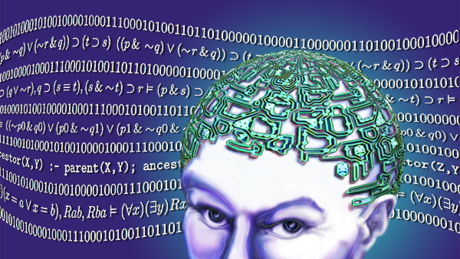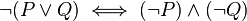# SatisfiabilitySatisfiability
http://en.wikipedia.org/wiki/Satisfiability

In mathematical logic, satisfiability and validity are elementary concepts of semantics.
A formula is satisfiable if it is possible to find an interpretation (model) that makes the formula true.
A formula is valid if all interpretations make the formula true.

The opposites of these concepts are unsatisfiability and invalidity, that is, a formula is unsatisfiable if none of the interpretations make the formula true, and invalid if some such interpretation makes the formula false.
These four concepts are related to each other in a manner exactly analogous to Aristotle’s square of opposition.

concept used by:
Logic: Language and Information 1
The University of Melbourne
https://www.coursera.org/course/logic1

# De Morgan’s lawsDe Morgan’s laws
http://en.wikipedia.org/wiki/De_Morgan’s_laws

In propositional logic and boolean algebra, De Morgan’s laws are a pair of transformation rules that are both valid rules of inference.
The rules allow the expression of conjunctions and disjunctions purely in terms of each other via negation.

The rules can be expressed in English as:
The negation of a conjunction is the disjunction of the negations.
The negation of a disjunction is the conjunction of the negations.

or informally as:
“not (A and B)” is the same as “(not A) or (not B)”
and also,
“not (A or B)” is the same as “(not A) and (not B)”

The rules can be expressed in formal language with two propositions P and Q as:

Text Searching

De Morgan’s laws commonly apply to text searching using Boolean operators AND, OR, and NOT.
Consider a set of documents containing the words “cars” or “trucks”.
De Morgan’s laws hold that these two searches will return the same set of documents:
Search A: NOT (cars OR trucks)
Search B: (NOT cars) AND (NOT trucks)

# ex falso quodlibetPrinciple of explosion
http://en.wikipedia.org/wiki/Principle_of_explosion

The principle of explosion, (Latin: ex falso quodlibet, “from a falsehood, anything follows”, or ex contradictione sequitur quodlibet, “from a contradiction, anything follows”) or the principle of Pseudo-Scotus, is the law of classical logic, intuitionistic logic and similar logical systems, according to which any statement can be proven from a contradiction.
That is, once a contradiction has been asserted, any proposition (or its negation) can be inferred from it.

cited on:
Logic: Language and Information 1
The University of Melbourne
https://www.coursera.org/course/logic1

# Lexical ambiguityScope and ambiguity in expanded categorial semantics
http://people.umass.edu/gmhwww/595/pdf/book/!10-Scope.pdf

Ambiguity:
The traditional view is that ambiguity comes in two varieties – lexical ambiguity, and structural ambiguity.

Lexical ambiguity:
In instances of lexical ambiguity, a single morpheme has two or more entries in the lexicon;  alternatively, the same surface form (spelling/pronunciation) corresponds to two or more morphemes. A
prominent logical example is ‘and’ which has two different meanings, as in:

• Jay respects Kay and Elle
• Jay is between Kay and Elle

For example, the first one means that Jay respects Kay and Jay also respects Elle, but the second one does not mean that Jay is between Kay and Jay is between Elle, which is nonsense.

=================

Introduction to Natural Language Processing
University of Michigan
Coursera, October 5 – December 27, 2015
https://www.coursera.org/course/nlpintro

Natural Language Processing (2013)
by Michael Collins
University of Columbia
https://class.coursera.org/nlangp-001/wiki/syllabus

# Gödel’s incompleteness theoremsGödel’s incompleteness theorems
http://en.wikipedia.org/wiki/G%C3%B6del’s_incompleteness_theorems

The theorems, proven by Kurt Gödel in 1931, are important both in mathematical logic and in the philosophy of mathematics.

For any such system, there will always be statements about the natural numbers that are true, but that are unprovable within the system.

The second incompleteness theorem, an extension of the first, shows that such a system cannot demonstrate its own consistency.

The liar paradox is the sentence “This sentence is false.” An analysis of the liar sentence shows that it cannot be true (for then, as it asserts, it is false), nor can it be false (for then, it is true). A Gödel sentence G for a theory T makes a similar assertion to the liar sentence, but with truth replaced by provability: G says “G is not provable in the theory T.”

Extensions of Gödel’s original result
… it is common to state the effectiveness and expressiveness conditions as hypotheses for the incompleteness theorem, so that it is not limited to any particular formal theory. The terminology used to state these conditions was not yet developed in 1931 when Gödel published his results.

the epistemological relevance of the second incompleteness theorem

Examples of undecidable statements
There are two distinct senses of the word “undecidable” in mathematics and computer science. The first of these is …

Limitations of Gödel’s theorems
The conclusions of Gödel’s theorems are only proven for the formal theories that satisfy the necessary hypotheses.
Not all axiom systems satisfy these hypotheses, …

Gödel’s theorems only apply to effectively generated (that is, recursively enumerable) theories.
If all true statements about natural numbers are taken as axioms for a theory, then this theory is a consistent, complete extension of Peano arithmetic (called true arithmetic) for which none of Gödel’s theorems apply in a meaningful way, because this theory is not recursively enumerable.

Minds and machines
Authors including J. R. Lucas have debated what, if anything, Gödel’s incompleteness theorems imply about human intelligence. Much of the debate centers on whether the human mind is equivalent to a Turing machine, or by the Church–Turing thesis, any finite machine at all. If it is, and if the machine is consistent, then Gödel’s incompleteness theorems would apply to it.

# Schaum’s Outline of LogicSchaum’s Outline of Logic, Second Edition
January 27, 2011
http://www.mhprofessional.com/product.php?cat=145&isbn=0071755462

1. Argument Structure
2. Argument Evaluation
3. Propositional Logic
4. The Propositional Calculus
5. The Logic of Categorical Statements
6. Predicate Logic
7. The Predicate Calculus
8. Fallacies
9. Induction
10. The Probability Calculus
11. Further Developments in Formal Logic

# Logic is hard, mathematics harder, and probability even more challenging

Humans find logic hard, mathematics harder, and probability even more challenging*.
Psychologists have discovered systematic violations of probability calculations and behavior by humans.
Consider, for example, the Monty Hall problem https://franzcalvo.wordpress.com/2013/11/08/monty-hall-problem.

*bibliographic references are not provided for this statement.